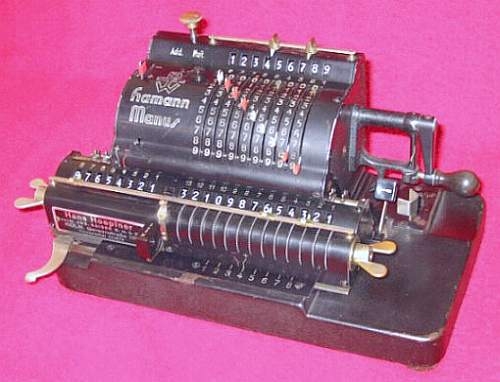previous <<==>> next

HAMANN-MANUS   » C «``` The broken plastic LEVER CAPS are replaced by colored insulator pipes README the New Model Identification ... T E C H N I C A L D A T A of the Mechanical Calculator HAMANN-Manus *********************************************************************** ( New Identification ) Model » C2 « Serial Number: 6229 Dimensions: (ca.) Width = 10_1/4" / 26 cm Depth = 6 " / 15 cm Height = 6 " / 15 cm Weight: (ca.) 12 lbs / 5.5 kg Mechanics: Switching-Latch-Wheel / Schalt-Klinke Functions: Add, Subtract, Multiply, Divide AUTOMATED DIVISION ! Registers: Input = 9 Decimals Counter = 8 Decimals Arithmetic = 13 Decimals Manufacturer: DTW ==>> DeTeWe Deutsche Telephon Werke AG Berlin / Germany 1930 H O W T O U S E the HAMANN-Manus » C2 « ************************************************ BASIC SETTINGS: =============== (A) Crank: ---------- In the idle position the crank is down and locked. To make one or more turns (clockwise only!) with the crank, pull out the handle to unlock. When finished the turn(s), let the handle snap in the lock. The locked down position only makes other functions (ex. clearing) accessible. REMARKS: Every started turn has to be finished completely! ******** Accidentally started turns are correctible somehow. The little "WINDOW" between Counter and Arithmetic Unit indicates OVER-/UNDERFLOW and has to be reset manually. The little lever under the name » HAMANN / MANUS « is only for "EMERGENCY" when the crank is blocked: Pulling the lever allows to crank backward. (B) Clear Input Unit: --------------------- Press the (->) knob on top to clear the input unit. When the knob is turned either to (Add <- ), than the input unit will be cleared after an operation, or turned to ( -> Mult), than the input unit will not be cleared after an operation. (C) Clear Counter & Arithmetic Unit in the Carriage: ---------------------------------------------------- Press-Down & Hold the [:/x] lever to shift the carriage to the far left position. This position is for clearing (and initial settings) only - No arithmetic operations are possible in this position! Turning the right wing nut will clear counter & arithmetic unit simultaniously. With the left wing nut the counter unit only will be cleared. When pressing the metal "tongue" (in the middle) while turning the right wing nut, only the arithmetic unit will be cleared. Only in this position it is possible to pre-set the arithmetic unit with the affiliated toothed wheels (13 ... 1). (D) Set the Carriage in Start Position 1: ----------------------------------------- Push the big left horizontal lever on front one time to the right. Pull the [:/x] front lever to [x]. (E) Set Counting Direction with the [+/-] Lever on Top to [+] ------------------------------------------------------------- In this case counter and arithmetic unit are working in the same direction; In case of [-] both are working opposit direction. (F) Set the [Add/Sub] lever (under crank) to [Add]: --------------------------------------------------- This will set the carriage / arithmetic unit in [+] state; If set to [Sub], the carriage / arithmetic unit would be set in [-] state. ( The crank is turnable clockwise only! ) ADDITION & SUBTRACTION: ======================= Example: 123 + 45 - 6 = 162 ADD: Enter the first number (123) in the far right of the input unit. Make a (clockwise only!) turn with the crank to transfer input into arithmetic unit. The counting unit displays the figure 1. Enter the second number (45) into input unit. Turn the crank to add the number. The arithmetic unit displays the intermediate sum (168) and the counting unit displays the figure 2. SUBTRACT: Switch [Add/Sub] lever to [Sub]. Enter the third number (6) into input unit. Make a (clockwise only!) turn with the crank. The arithmetic unit displays the result (162) and the counting unit is decreased by 1. REMARK: NEGATIVE RESULTS are displayed in the arithmetic unit as the COMPLEMENT of the next higher 10, 100, 1000, ... Example: -12 = 99...9988 MULTIPLICATION: =============== Example: 123 x 45 = 5535 Clear registers and set (Add/Mult) knob to ( -> Mult). Enter the multiplicand (123) in the far right of the input unit. The multiplicator (45) has two digits, so the arithmetic unit is set to position 2 with a push on the big left horizontal lever on front. Make (clockwise only!) turns with the crank, until the first figure of the multiplicator (4) will appear in the 2nd position of the counter unit. Move the arithmetic unit to position 1 by pressing the arrow [<-] key. Repeat making turns with the crank, until the second figure of the multiplicator (5) appears in the 1st position of the counter unit. The multiplication is done: The multiplicand (123) stays in the input unit, the multiplicator (45) is in the counter unit and the result (5535) is in the arithmetic unit. DIVISION: ========= Example: 22 : 7 = 3.1428571 Remainder 3 Set the (Add/Mult) knob to ( -> Mult); set the [Add/Sub] lever to [Sub]. In the "Basic Setting Position" (after clearing counter & arithmetic unit) set the dividend (22) in the far left of the arithmetic unit with the affiliated toothed wheels. Shift the carriage in position 8 and push the [:/x] lever to [:]. Set the divisor (7) into input unit above the dividend (22). Set Counting Direction Lever on Top to [-]. To devide make continuous (clockwise!) turns with the crank, until the division is finished. The algorithm (Subtraction until underflow, Add, Shift carriage, Continue...) is done automatically! The result (3.1428571) can be seen in the counter unit, and the remainder (3) is in the arithmetic unit. The divisor (7) is in the input unit, therefore an additional decimal can be estimated... ( 4, cause 4 x 7 = 28 ). Have a look at "Calculating Trickies" ... impressum: *********************************************************************** © C.HAMANN http://public.BHT-Berlin.de/hamann 12/15/07 ```